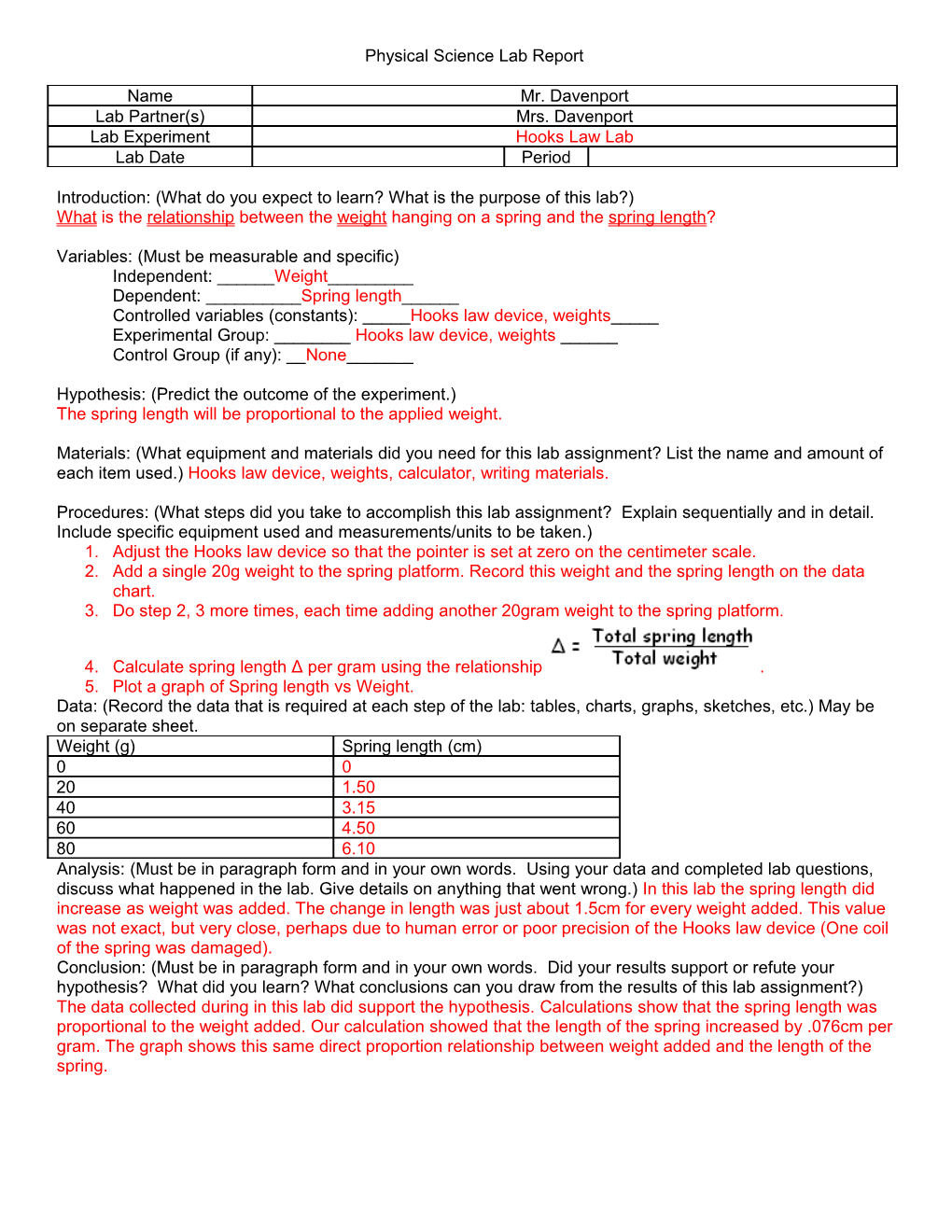# Physical Science Honors Lab ReportPhysical Science Lab Report

Name / Mr. Davenport
Lab Partner(s) / Mrs. Davenport
Lab Experiment / Hooks Law Lab
Lab Date / Period

Introduction: (What do you expect to learn? What is the purpose of this lab?)

What is the relationship between the weight hanging on a spring and the spring length?

Variables: (Must be measurable and specific)

Independent: ______Weight______

Dependent: ______Spring length______

Controlled variables (constants): _____Hooks law device, weights_____

Experimental Group: ______Hooks law device, weights ______

Control Group (if any): __None______

Hypothesis: (Predict the outcome of the experiment.)

The spring length will be proportional to the applied weight.

Materials: (What equipment and materials did you need for this lab assignment? List the name and amount of each item used.) Hooks law device, weights, calculator, writing materials.

Procedures: (What steps did you take to accomplish this lab assignment? Explain sequentially and in detail. Include specific equipment used and measurements/units to be taken.)

1. Adjust the Hooks law device so that the pointer is set at zero on the centimeter scale.
2. Add a single 20g weight to the spring platform. Record this weight and the spring length on the data chart.
3. Do step 2, 3 more times, each time adding another 20gram weight to the spring platform.
4. Calculate spring length Δ per gram using the relationship .
5. Plot a graph of Spring length vs Weight.

Data: (Record the data that is required at each step of the lab: tables, charts, graphs, sketches, etc.) May be on separate sheet.

Weight (g) / Spring length (cm)
0 / 0
20 / 1.50
40 / 3.15
60 / 4.50
80 / 6.10

Analysis: (Must be in paragraph form and in your own words. Using your data and completed lab questions, discuss what happened in the lab. Give details on anything that went wrong.)In this lab the spring length did increase as weight was added. The change in length was just about 1.5cm for every weight added. This value was not exact, but very close, perhaps due to human error or poor precision of the Hooks law device (One coil of the spring was damaged).

Conclusion: (Must be in paragraph form and in your own words. Did your results support or refute your hypothesis? What did you learn? What conclusions can you draw from the results of this lab assignment?)

The data collected during in this lab did support the hypothesis. Calculations show that the spring length was proportional to the weight added. Our calculation showed that the length of the spring increased by .076cm per gram. The graph shows this same direct proportion relationship between weight added and the length of the spring.

Page 2: Calculations:

Page 3: Graph